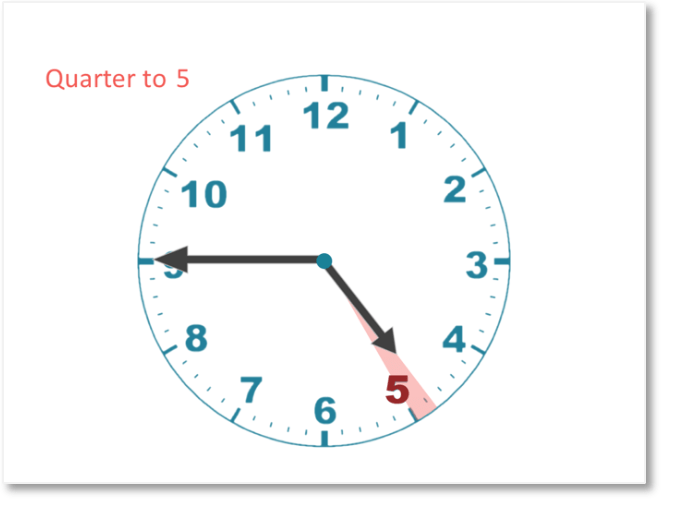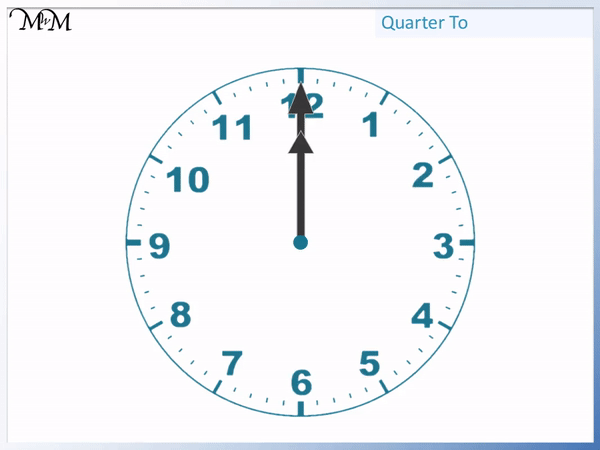# How to Tell the Time to a Quarter to the Hour

How to Tell the Time to a Quarter to the Hour• When the longer minutes hand is pointing at the 9, there is one quarter of an hour to go until the next hour.
• When the minutes hand is pointing at the 9, it is a quarter to the next hour.
• The minute hand needs to travel another quarter of the clock to get to the next hour.
• The shorter hour hand is between the 1 and the 2 and so, the next hour is 2 o’clock.
• The time shown is a quarter to 2.
• This means that it is fifteen minutes until 2 o’clock.
• Because 45 minutes have passed since 1 o’clock, the time can also be written as 1:45.
• It is 15 minutes until 2 o’clock.

If the minutes hand is pointing at the 9, then the time is a quarter to the hour.

The number that the hour hand will reach next tells us which hour this is.• After 4 o’clock, the minute hand moves around to point at the 9.
• Since the minute hand is pointing at the 9, the time is a quarter to the next hour.
• We have already had 4 o’clock and the next hour is 5 o’clock.
• We can see that the next number that the hour hand will point at is a 5.
• The time is a quarter to 5.
• The time can also be written as 4:45 since it is 45 minutes past 4 o’clock.# Telling the Time: Quarter to the Hour

## What is Quarter to the Hour?

A quarter to the hour is when it is 15 minutes until the next hour. We know it is a quarter to the hour when the minute hand is pointing at the 9.

Here are the two hands of a clock. The longer hand is the minute hand and the shorter hand is the hour hand.When the minute hand completes a full turn, an hour has passed. From one hour to the next, the minute hand rotates from pointing at the 12 all the way around to point back at the 12 again.

With any time we can say how many minutes it is past the previous hour or we can say how many minutes it will be until the next hour.

When the minute hand reaches the 6 at the bottom, it is halfway around the clock.Before the minute hand reaches the bottom it is nearer to the previous hour and so, we use minutes past the hour.

After the minute hand reaches the bottom, it is now nearer to the next hour. It is now easier to say how many minutes it is until the next hour instead of how many minutes past the previous hour it is.When the minute hand is pointing directly at the 9, there is one-quarter of an hour to go until the next hour.

In this example, the next hour is 2 o’clock.It is easier to say that the time is a quarter to 2 instead of saying that it is 45 minutes past one.

Because the time is close to 2 o’clock, it is more helpful to say a quarter to 2 as it reminds us that it is almost 2 o’clock. We can see that the hour hand is one-quarter of the way away from pointing at the 2.For a quarter to the hour, the minute hand is pointing exactly at 9 but the hour hand is in between two numbers. The larger of these numbers is the hour that it is a quarter to.An hour is 60 minutes long. A quarter of 60 is 15. So when it is a quarter to the next hour, it is 15 minutes to the next hour.

45 minutes have already passed since the previous hour so all times that are quarter to the next hour are the same as 45 minutes past the previous hour.

When written down, times that are a quarter to the next hour will always display 45 minutes past the previous hour. For example, 1:45 is a quarter to 2 and 6:45 is a quarter to 7.

We cannot write a quarter to the hour in the digital clock form. We have to write the previous hour, put the colon and then a 45.

## How to Show Quarter to the Hour on a Clock

When the minute hand is pointing directly at the 9, it is quarter to the next hour. Look at the next number that the hour hand will reach to see what this next hour is.

Here is an example of reading quarter to when telling the time.The minute hand is pointing directly at the 9 and so, we know the time is quarter to the next hour.

We look at the shorter hour hand and see that the next number it will reach is 5. So the time is a quarter to 5.

We can see that the hour hand is almost pointing at the 5 but just not quite there. When it finally reaches 5 o’clock, then the hour hand will eventually point exactly at the 5.Quarter to 5 can also be said as ‘one forty-five’ and written as 1:45.

## How to Teach Quarter to the Hour

To teach quarter to the hour, it is first important to understand how to read quarter past the hour. Then it is worth noticing the pattern that all times that are quarter to the hour will have a minute hand pointing directly at the 9.

It is also helpful to point out when it is quarter to the next hour in day to day life and also to encourage your child to try and do the same.

Here is a quarter to 9.

When teaching quarter past and quarter to, it is important to focus on the hour hand to make sure they know which number to read.

The hour hand is between 8 and 9.

Either it is 8:45 or a quarter to 9. It is worth looking at both answers and showing how this relates to the position of the hour hand.In general, you should choose the number that the hour hand is closest to when reading quarter past or quarter to.It can also be helpful to show diagrams like those shown on this page, by drawing a clock face and shading it in. This can help explain quarters and why when the minute hand points at 3, it is quarter past and when the minute hand points at 9, it is quarter to.

Here is another example.The minute hand is pointing at the 9 and so, it is a quarter to the next hour. We look at the hour hand and see that the next hour it reaches is 11.

The time is a quarter to 11.Now try our lesson Telling the Time to 5 Minute Intervals where we learn how to tell the time to 5 minute intervals.